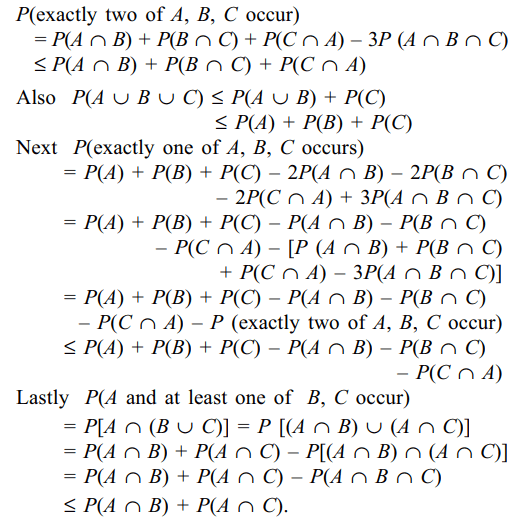## Probability Questions and Answers Part-5

1. A group of 6 boys and 6 girls is randomly divided into two equal groups. The probability that each group contains 3 boys and 3 girls is
a) 10/231
b) 5/231
c) 90/231
d) 100/231

Explanation: The number of ways of choosing 6 persons out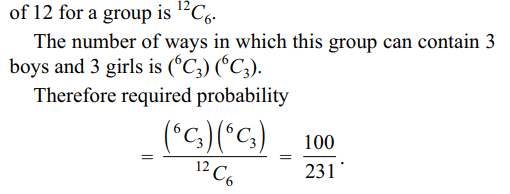2. In a hurdle race, a runner has probability p of jumping over a specific hurdle . Given that in 5 trials, the runner succeeded 3 times, the conditional probability that the runner had succeeded in the first trial is
a) $\frac{3}{5}$
b) $\frac{2}{5}$
c) $\frac{1}{5}$
d) $\frac{4}{5}$

Explanation: Let A denote the event that the runner succeeds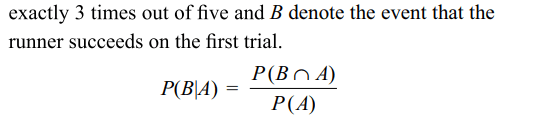3. Let F denote the set of all onto functions from $A=\left\{a_{1},a_{2},a_{3},a_{4}\right\}$     to $B=\left\{ x,y,z,\right\}$    . A function f is chosen at random from F. The probability that f–1{x} consists of exactly one element is
a) 2/3
b) 1/3
c) 1/6
d) 0

Explanation: Let us first count the number of elements in F4. Three integers are chosen at random without replacement from the first 20 integers. The probability that their product is even is
a) 7/19
b) 2/19
c) 17/19
d) 10/19

Explanation: The product of any number of integers will be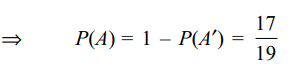5. A box contains tickets numbered 1 to N. n tickets are drawn from the box with replacement. The probability that the largest number on the tickets is k is
a) $\left(\frac{k}{N}\right)^{n}$
b) $\left(\frac{k-1}{N}\right)^{n}$
c) 0
d) $\frac{k^{n}-\left(k-1\right)^{n}}{N^{n}}$

Explanation: Let X denote the largest number on the n tickets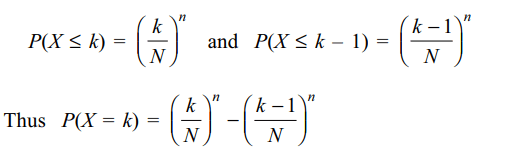drawn.

6. A biased cubical die is thrown twice. Let
P = probability of getting the same number both the times.
Q= probability getting odd number on the first thrw and an even number on the second throw.
Then
a) $Q \leq\frac{1}{4}$
b) $P \geq\frac{1}{3}-\frac{2}{3}Q$
c) $P +2Q\leq 1$
d) All of the Above

Explanation: Let pi = probability of getting number i on the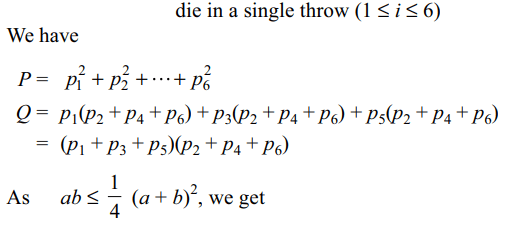7. An unbiased cubical die is thrown 2n times, and let P denote the probability of getting even numbers at least n times, then
a) $P >\frac{1}{2}$
b) $P \geq\frac{1}{2}+\frac{1}{4n}$
c) $P =\frac{163}{256}$   if n=4
d) All of the Above

Explanation: Let p = probability of getting an even number on a single throw of the die
and let X = number of times an even number is obtained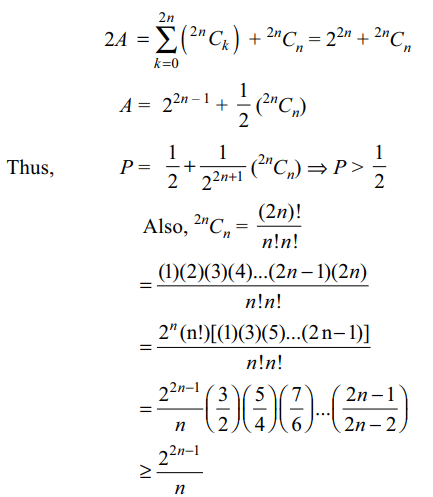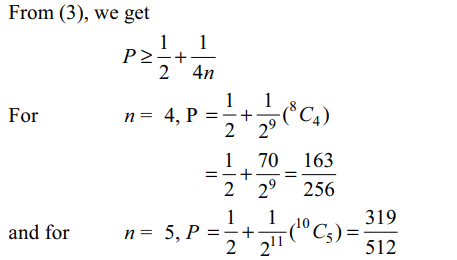8. If A and B are two events, the probability that exactly one of them occurs is given by
a) $P\left(A\right)+P\left(B\right)-2P\left(A\cap B\right)$
b) $P\left(A\cap B'\right)+P\left(A'\cap B\right)$
c) $P\left(A\cup B\right)-P\left(A\cap B\right)$
d) All of the Above

Explanation: We have P(exactly one of A, B occurs)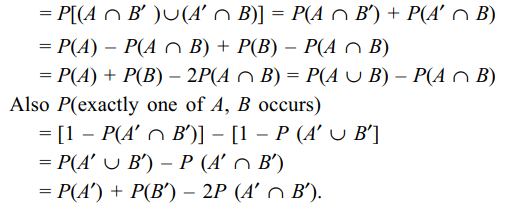9. If A and B are two events such that P(A) = 1/2 and P(B) = 2/3, then
a) $P\left(A\cup B\right)\geq 2/3$
b) $P\left(A\cap B'\right)\leq 1/3$
c) $1/16\leq P\left(A\cap B\right)\leq 1/2$
d) All of the Above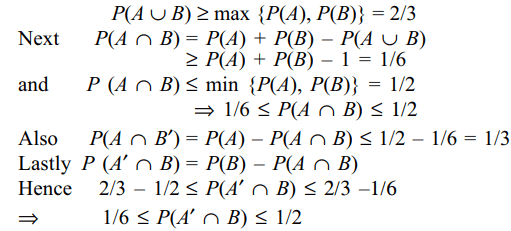a) P (exactly two of A, B, C occur) $\leq P\left(A \cap B\right) + P\left(B \cap C\right) + P\left(C \cap A\right)$
b) $P\left(A\cup B \cup C\right) \leq P\left(A\right) + P\left(B\right) + P\left(C\right)$
c) P (exactly one of A, B, C occur) $\leq P\left(A\right) + P\left(B\right) + P\left(C\right) – P\left(B \cap C\right) – P\left(C\cap A\right) – P\left(A \cap B\right)$